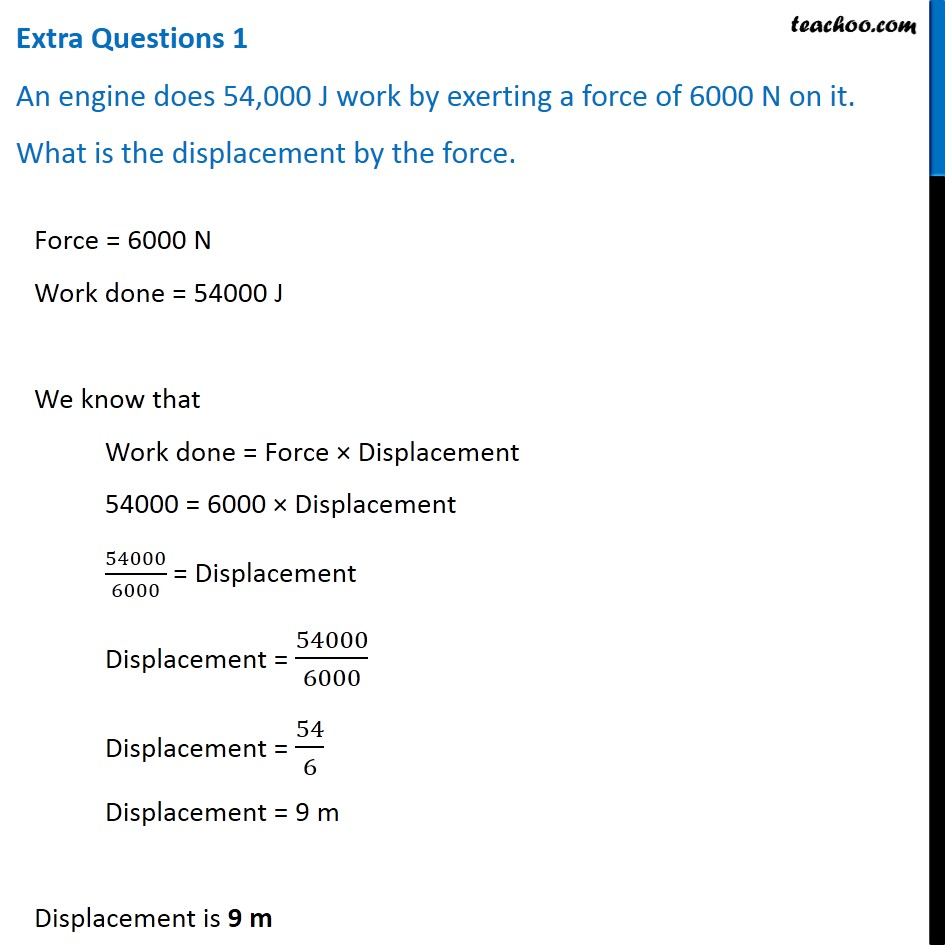Teachoo Questions

Class 9
Chapter 11 Class 9 - Work and EnergyGet live Maths 1-on-1 Classs - Class 6 to 12

### Transcript

Extra Questions 1 An engine does 54,000 J work by exerting a force of 6000 N on it. What is the displacement by the force. Force = 6000 N Work done = 54000 J We know that Work done = Force × Displacement 54000 = 6000 × Displacement 54000/6000 = Displacement Displacement = 54000/6000 Displacement = 54/6 Displacement = 9 m Displacement is 9 m Displacement = 9 m Displacement is 9 m